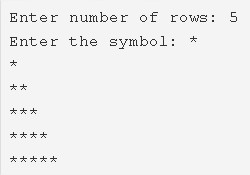# C Program Right Triangle Star Pattern | Pattern Programs

C program to print a right triangle star pattern – In this article, we will brief in on the different means to print a right triangle star pattern in C programming.

Suitable examples and sample programs have also been added so that you can understand the whole thing very clearly. The compiler has also been added with which you can execute it yourself.

The following program is written in three different ways:

• Using For Loop
• Using While Loop
• Using Do-While Loop

It is not a new thing to the world of coders what a Right Triangle is. It is a type of a triangle which has one angle equal to 90 degrees.

The sum of squares of the sides adjacent to the right angle is equal to the square of the side opposite to the right angle.Firstly, as per the given example, you need to certain the number of rows of the pattern to be printed.

The number of rows specified for this pattern in the example is 5.

Then, you need to particularise the symbol you need to use to print the pattern.

The symbol ‘*’ is chosen to do the honours as per the name of the title itself.

Hence, the pattern is printed subsequently as per the code.

Thus, the different means to do the same in C programming are as follows:

## Using For Loop

1. Read the number of rows and store that value into the variable n, store the symbol into the variable ch which is entered by the user.

2) The outer for loop iterates through rows from i=1 to n with the structure for(i=1;i<=n;i++).

3) The inner for loop iterates through columns with the structure for(j=0;j<i;j++).

a) Prints symbol.

4) Cursor comes to next line.

5) Each row contains that row number of symbols.

6) In given example n=5.

At 1st row the inner loop prints one star, at 2nd row it prints 2 stars, at 3rd row, it prints 3 stars, at 4th row, it prints 4 stars, at 5th row, it prints 5 stars. Then we will get right triangle star pattern with 5 rows.

Output:

### Using While Loop

1. The outer while loop iterates through rows until the condition i<=n becomes false.

2) The inner while loop iterates through columns until the condition j<i becomes false.

a) Prints symbol.

b) j value increased by 1.

3) Cursor comes to next line.

4) i value increased by 1. The outer while loop iterates until the condition becomes false.

Output:

#### Using Do-While Loop

1. The outer do-while loop iterates through rows.

2) The inner do-while loop iterates through columns.

a) Prints symbol.

b) j value increased by 1.

c) Checks the condition j++<n. If the condition is true then loop iterates again. Repeats until the condition becomes false.

3) Cursor comes to next line.

4) i value increased by 1.

5) Checks the condition ++i<n. If the condition is true then the inner loop iterates again. Repeats until the condition becomes true.

Output:

x

## C Program To Check If Alphabet, Digit or Special Character | C Programs

C program to check for an alphabet, digit or special character – In this article, ...